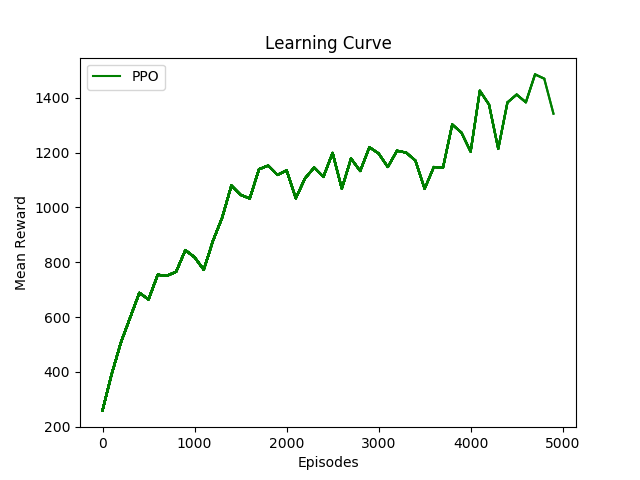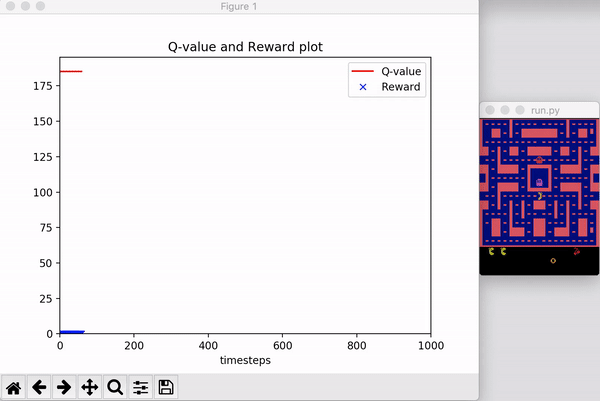# NIUHE

### RL - Proximal Policy Optimization (PPO)

Proximal Policy Optimization (PPO, PPO-Clip, PPO-Penalty) 是由TRPO的作者Schulman等人于2017年提出的策略梯度类算法。PPO算法的思路和TRPO一致，都是想在优化时采取尽可能大的步幅但又不能太大以至于产生崩坏。相比于比TRPO，PPO实现起来更简单，泛化能力更强，可以使用随机梯度下降（SGD）进行优化。

## 背景

PPO的背景与TRPO的背景一致，最终TRPO推导出如下的带约束优化问题： $\max_{\theta}\mathbb{E}_t[\frac{\pi_\theta(a_t|s_t)}{\pi_{\theta_{old}}(a_t|s_t)}A_t]\\ \text{subject to }\mathbb{E}_t[\text{KL}[\pi_{\theta_{old}}(\cdot|s_t), \pi_\theta(\cdot|s_t)]]$$r_t(\theta) = \frac{\pi_\theta(a_t|s_t)}{\pi_{\theta_{old}}(a_t|s_t)}$ 为新旧策略的概率比（易知 $r_t(\theta_{old}) = 1$）。TRPO最大化的替代目标（surrogate objective）可以写为如下形式： $L^{CPI}(\theta)=\mathbb{E}_t[\frac{\pi_\theta(a_t|s_t)}{\pi_{\theta_{old}}(a_t|s_t)}A_t]=\mathbb{E}_t[r_t(\theta)A_t]$ 如果不加约束的话，直接优化该目标会产生巨大的更新，导致更新不稳定甚至崩溃。所以需要考虑一种惩罚方法，使 $r_t(\theta)$ 接近 $1$

## PPO-Clip

PPO-Clip的目标函数为： $L^{CLIP}(\theta)=\mathbb{E}_t[\min(r_t(\theta)A_t, \text{clip}(r_t(\theta), 1-\epsilon, 1+\epsilon)A_t)]$ 其中 $\epsilon$ 为超参数控制截断率，取值通常比较小（0.2左右）。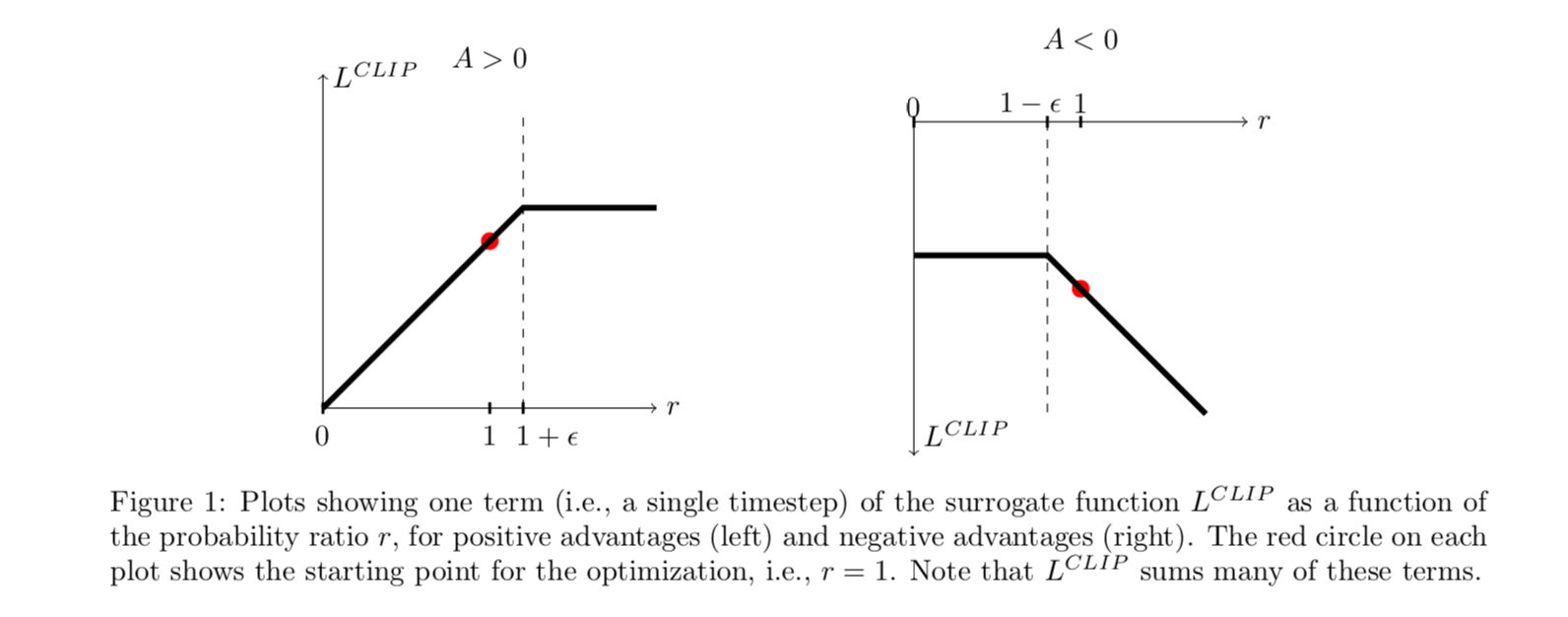• $A > 0$ 时，如果想使目标函数取得更大的值，就需要增大 $\pi_\theta(a_t|s_t)$ 的值，也就是增大 $r_t(\theta)$ 。但是式中的 $\min$ 函数限制了 $r_t(\theta)$ 最大取到 $1+\epsilon$，所以新策略再远离旧策略（$r_t(\theta)$ 继续增大）并不会带来更多地好处。
• $A < 0$ 时，如果想使目标函数取得更大的值，就需要减小 $\pi_\theta(a_t|s_t)$ 的值，也就是减小 $r_t(\theta)$ 。但是式中的 $\min$ 函数限制了 $r_t(\theta)$ 最小取到 $1-\epsilon$，所以新策略再远离旧策略（$r_t(\theta)$ 继续减小）并不会带来更多地好处。

PPO-Clip算法## PPO-Penalty

• 使用SGD优化目标函数： $L^{KLPEN}(\theta)=\mathbb{E}_t\left[\frac{\pi_\theta(a_t|s_t)}{\pi_{\theta_{old}}(a_t|s_t)}A_t-\beta\cdot\text{KL}[\pi_{old}(a_t|s_t), \pi_\theta(a_t|s_t)]\right]$

• 计算 $d = \mathbb{E}\left[\text{KL}[\pi_{\theta_{old}}(\cdot|s_t), \pi_\theta(\cdot|s_t)]\right]$

• 如果 $d < d_{targ}/1.5$，则 $\beta \leftarrow \beta/2$
• 如果 $d > d_{targ} \times 1.5$，则 $\beta \leftarrow\beta \times 2$

其中，$d_{targ}$ 为超参数。

## 实验和总结

• 训练稳定
• 通过限制 $r_t(\theta)$ 来找到尽可能大的并且合理的步长
• on-policy 算法
• 可用于离散和连续的动作空间
• 相比于TRPO，PPO实现简单，效果更好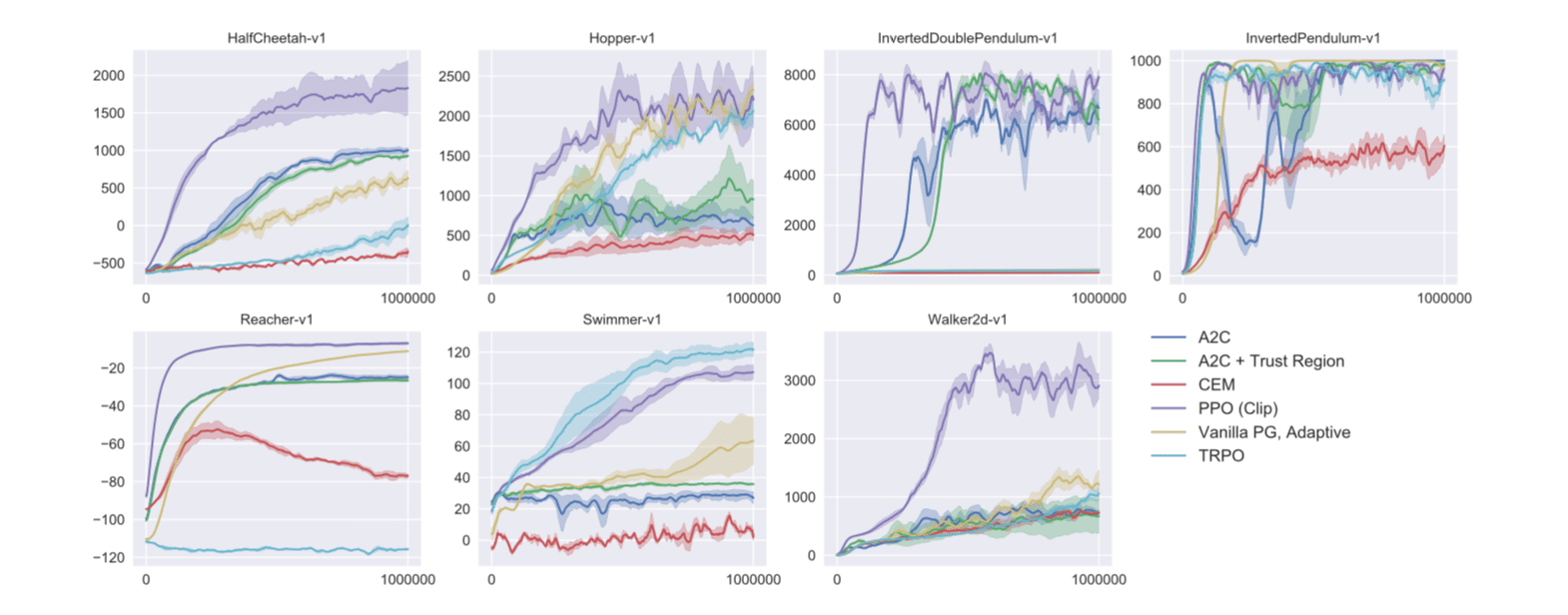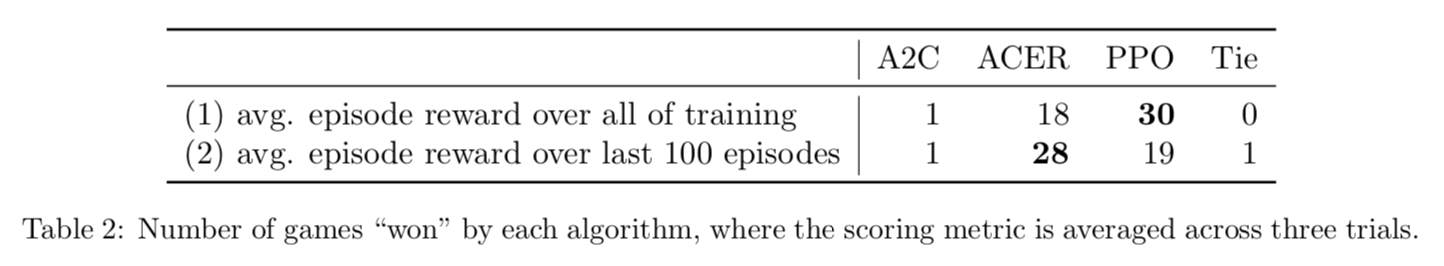### 代码